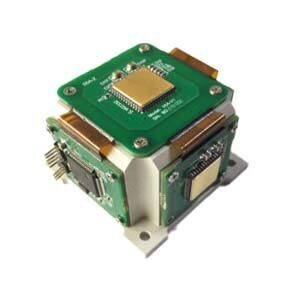Application

# What does an IMU Stand for?What are 6-axis, 9-axis, IMU?

6-axis: three-axis (XYZ) accelerometer + three-axis (XYZ) gyroscope (also known as angular velocity sensor), the module is powered on each heading angle is 0, there is no absolute reference (east, west, north and south). Cannot be used as a compass; Speaking of 6-axis, for example, ER-MIMU-01 North-Seeking MEMS IMU is a self-developed 6-axis MEMS IMU with built-in gyroscope and accelerometer, which can measure linear acceleration and rotational angular velocity from three directions, and obtain the attitude, speed and displacement information of the carrier through analysis. It is widely used in oil exploration. Bridges, high-rise buildings, towers, dam monitoring, geotechnical monitoring, mining and other fields.

9 axis: 6 axis + 3 axis (XYZ) magnetic field sensor. If the calibration is completed, then the heading Angle has an absolute value (there are east, west, north and south) each time it is powered on, and can be used as a compass. However, the 9-axis mode requires calibration and is easy to receive magnetic interference (basically not used indoors).

IMU: Inertial Measurement Unit (IMU) is a device that measures the triaxial angular velocity and acceleration of an object. An IMU may contain a three-axis gyroscope and a three-axis accelerometer to measure the angular velocity and acceleration of an object in three dimensions. Strictly IMU only provides users with triaxial angular velocity and triaxial acceleration data.

IMU can measure (output) what data

IMU = accelerometer + angular velocimeter (gyroscope), so of course you can measure (output) acceleration and angular velocity (equivalent to the direct output of the sensor on the module). In addition, the processing unit on the module can also use acceleration and angular velocity integration to obtain the angle (Euler angle, or attitude) and linear velocity, but the linear velocity is generally not output, because the linear velocity error obtained by acceleration integration is very large and diverge with time (if it has been moving for a few minutes, it will be too large to make any sense). As for the displacement (speed continues to integrate) error will be more exaggerated (integral once, error accumulates once). In terms of output data, ER-MIMU-06 High Precision Navigation MEMS IMU can output the original hexadecimal complement data of X, Y, Z three-axis gyroscope and accelerometer through RS422 (including the numerical temperature and angle of the gyroscope hexadecimal complement, the accelerometer hexadecimal temperature, and the accelerometer hexadecimal complement number); It can also output the floating dimensionless values of the gyroscope and accelerometer processed by the underlying calculation.

When measuring attitude, passing through a gyroscope is sufficient. Why do we need such a complex IMU to accomplish this?

The gyroscope is very good at measuring the attitude in a short time, and the direction is calculated by integrating the angular velocity of the gyroscope. If only the gyroscope measurement results are not perfect, it contains a certain error, this error is caused by the gyroscope output is not perfect, in order to eliminate this error, we add accelerometers and magnetometer sensors from the gyroscope to stabilize the reading.

Without professional equipment such as turntable, how to easily and quickly qualitatively evaluate the dynamic accuracy of IMU?

(Here is qualitative analysis, can not give quantitative results): a simple qualitative analysis method: place the module horizontally, pick up the module after stability for random maneuvering movement (slowly, not too intense, do not exceed the scope of the gyro), move for a certain time (2-5min) back to the horizontal position, this time you will find that the pitch roll angle has a “back” process. This is because the motion accelerometer no longer measures only the gravity vector, so it can not provide an absolute reference of the pitch roll angle, and can only rely on the gyroscopic integral to recurse the attitude, with the passage of time, the pure gyroscopic integral attitude will inevitably have errors. After being placed horizontally again, the module is at rest, and the accelerometer measures only the gravity vector, so it can continue to provide an absolute reference for the pitch roll Angle, hence the “righting” process. Therefore, the gyro performance of this product can be simply qualitatively compared from the size of the “correction” (rather than the speed). The greater the amplitude of correction, the greater the accumulated error of the gyroscope in the course of motion.

In addition, this “righting” process is difficult to see with the naked eye if only by looking at the pitch roll value, and must be observed by the upper computer using a curve or visual dashboard.